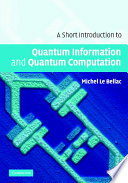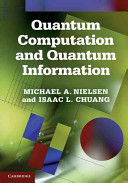### Quantum Computers

#### Course Description

Introduction to quantum mechanics with necessary elements of linear algebra. Dirac's notation. Classical vs. quantum bit and representation of the quantum bit state on the Bloch sphere. Quantum encription: quantum key distribution protocol BB84. Entanglement in quantum systems. Unitarity of quantum mechanics and time evolution of the quantum state. Quantum gates and algorythms: Deutsch algorithm, Shor factorisation algorithm, Grover search algorithm. Overview of candidate technologies for physical realisation of quantum computers: photon polarisation, nuclear magnetic resonance, ion traps, neutral atoms, Superconducting Josephson junction, quantum dots.

#### Learning Outcomes

1. explain the principles of quantum mechanics
2. apply Dirac notation in simple calculations in quantum mechanics
3. identify the state of the quantum bit on the Bloch sphere
4. describe the quantum key distribution protocol BB84
5. explain entanglement in a qantum systems
6. describe the Deutsch and Shor quantum algorythms
7. outline the basic features of candidate technologies for physical realization of quantum computers

#### Forms of Teaching

Lectures

Exercises

Partial e-learning

#### Week by Week Schedule

1. Introduction to quantum phenomena: polarization of light, Malus' law, probability, probability amplitude and interference, quantum bit (Qubit)
2. Example: Quantum key distribution protocol BB84
3. Principles of quantum mechanics: Representation of state by vectors in complex Hilbert space, Born's rule and measurement, unitarity of evolution, Scrhroedinger equation.
4. Matrix representation of states and operators
5. Representation of qubit state on Bloch's sphere, qubit realisation through projection of spin 1/2.
6. Composite systems: pure and mixed states, entanglement, state operator (density matrix)
7. Examples: teleportation, dense coding
8. Mid-term exam
9. Reversibility of quantum logical circuit and reversibility of classical logical gates
10. Quantum logic gates (operators): Pauli X, Y, and Z gates, R, S, and T-gate, Haddamard gate, SWAP operator, control-NOT gate and universal control gate, Toffoli and Fredkin gates, useful identities
11. Deutsch and Deutsch-Jozsa algorithms, implementation in IBM QX
12. Grover's algorithm for unstructured database search, implementation in IBM QX
13. Quantum Fourier transform and Shor's algorithm, implementation in IBM QX
14. Physical realisation of quantum cmputers: DiVicenzo criteria, photonic systems, superconducting Josephson junctions, quantum dots, nuclear magnetic resonance
15. Final exam

#### Study Programmes

[FER3-EN] Computing - study
Elective Courses (5. semester)
[FER3-EN] Electrical Engineering and Information Technology - study
Elective Courses (5. semester)

#### LiteratureMichel Le Bellac (2006.), A Short Introduction to Quantum Information and Quantum Computation, Cambridge University PressMichael A. Nielsen, Isaac L. Chuang (2010.), Quantum Computation and Quantum Information, Cambridge University Press
Saša Ilijić (.), Kvantna računala: fizika, informacija i algoritmi (skripta u pripremi),

#### General

ID 223355
Winter semester
5 ECTS
L3 English Level
L1 e-Learning
45 Lectures
0 Seminar
15 Exercises
0 Laboratory exercises
0 Project laboratory